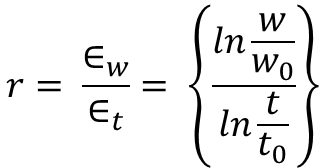## R-Value, The Plastic Anisotropy Ratio

The r-value, which is also called the Lankford coefficient or the plastic strain ratio, is the ratio of the true width strain to the true thickness strain at a particular value of length strain, indicated in Equation 1. Strains of 15% to 20% are commonly used for determining the r-value of low carbon sheet steel. Like n-value, the ratio will change depending on the chosen reference strain value.Equation 1

The normal anisotropy ratio (rm) defines the ability of the metal to deform in the thickness direction relative to deformation in the plane of the sheet. rm, also called r-bar (i.e. r average) is the “normal anisotropy parameter” in the formula for Equation 2:Equation 2

where r0, r90, and r45 are the r-value determined in 0, 90, and 45 degrees relative to the rolling direction.

For rm values greater than one, the sheet metal resists thinning. Values greater than one improve cup drawing (Figure 1), hole expansion, and other forming modes where metal thinning is detrimental. When For rm values are less than one, thinning becomes the preferential metal flow direction, increasing the risk of failure in drawing operations.Figure 1: Higher r-value is associated with improved deep drawability.

High-strength steels with tensile strength greater than 450 MPa and hot-rolled steels have rm values close to one. Therefore, conventional HSLA and Advanced High-Strength Steels (AHSS) at similar yield strengths perform equally in forming modes influenced by the rm value. However, r-value for higher strength grades of AHSS (800 MPa or higher) can be lower than one and any performance influenced by r-value would be not as good as HSLA of similar strength. For example, AHSS grades may struggle to form part geometries that require a deep draw, including corners where the steel is under circumferential compression on the binder. This is one of the reasons why higher strength level AHSS grades should have part designs and draw dies developed as “open ended”.

A related parameter is Delta r (∆r), the “planar anisotropy parameter” in the formula shown in Equation 3:Equation 3

Delta r is an indicator of the ability of a material to demonstrate a non-earing behavior. A value of 0 is ideal in can making or the deep drawing of cylinders, as this indicates equal metal flow in all directions, eliminating the need to trim ears prior to subsequent processing.

Testing procedures for determining r-value are covered in Citations A-44, I-15, J-14.

Related Posts
This month’s blog was contributed by

8

Citation U-13.

8

The Steel E-Motive program–commissioned by WorldAutoSteel in partnership with Ricardo plc–has developed the world’s f

8

You’ll find this content as part of our page on

8

Citation S-114 Courtesy of Schuler

8

Citation A-77. Published in the

8

Citation B-77. J. Born, “

8